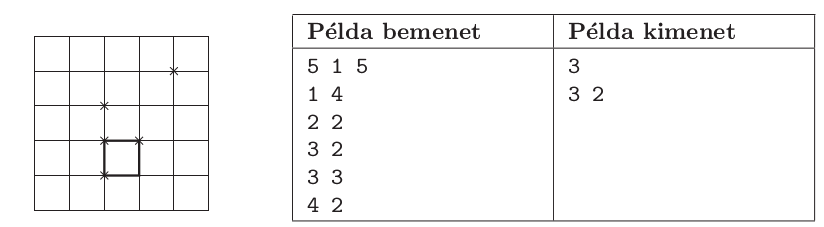Mathematical and Physical Journal
for High Schools
Issued by the MATFUND Foundation
 Already signed up? New to KöMaL?

#Problem S. 60. (February 2011)

S. 60. On a given square-shaped field we would like to fence off a smaller square with given area so that the little square contains as many trees as possible. The fences should be parallel to the sides of the original square, moreover, if a tree is situated just on the fence, it is considered to be inside''.

Your program should read the area of the original field and that of the little square, further, the position of the trees from the standard input, then give an optimal location for the little square on the standard output.

The first line of the input contains three numbers separated by a space: the side length ($\displaystyle 1 \le N \le 4000$) of the outer square-shaped field, the side length ($\displaystyle 1 \le M \le N$) of the little square to be fenced off, and the number of trees ($\displaystyle 1 \le K \le 1\;000\;000$). Then each of the next $\displaystyle K$ lines specifies the two coordinates (vertical, then horizontal) of a tree ($\displaystyle 0 \le I, J \le N$), separated by a space. Each coordinate is an integer, and no two trees have both coordinates in common.

The first line of the standard output should contain the number of captured trees within the small square, while the second line of the output should have the (vertical, then horizontal) coordinates of the upper left corner of the small square, separated by a space. If there are more solutions, any of them can be presented.

In the example, Példa bemenet'' is a sample input, while Példa kimenet'' is a possible output.

The time limit for your program is 10 seconds in each test case.

The source code and project files of your solution (without the .exe file or any other auxiliary files generated by the compiler) should be submitted together with a short documentation in a compressed folder.(10 pont)

Deadline expired on March 10, 2011.

Sorry, the solution is available only in Hungarian. Google translation

A feladatot dinamikus programozással lehetett megoldani. Mintamegoldásként Szabó Attila 10. osztályos pécsi tanuló megoldását közöljük. A kulcsgondolatot Borsos Zalán 12. osztályos marosvásárhelyi versenyző írta le a legvilágosabban (ábrát is mellékelve), így az ő dokumentációját is közzé tesszük.

### Statistics:

 9 students sent a solution. 10 points: Nagy 111 Miklós, Nagy Róbert, Szabó 928 Attila. 9 points: Borsos 607 Zalán. 5 points: 2 students. 4 points: 1 student. 2 points: 2 students.

Problems in Information Technology of KöMaL, February 2011